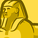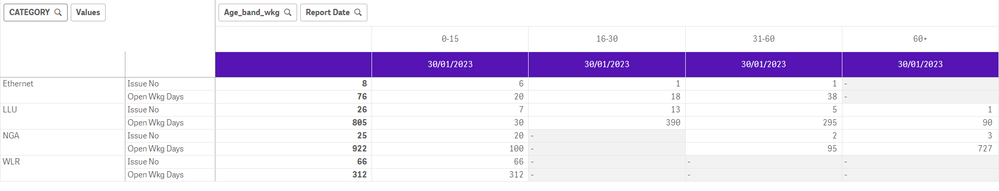Announcements
You can succeed best and quickest by helping others to succeed. Join the conversation.
cancel
Showing results for
Did you mean:Creator III

## Take the division of total values in Qliksense Pivot Table

Hello All ,

I have below pivot table in Qliksense:-Measure 1- Count(issue)  | Measure 2- sum(open_working_days)

I want to add one more column here named profile like below  and it should follow below calculation :-For Ethernet --- 76/8 = 9.50

LLU -- 806/26= 30.96 and so on

Labels (1)
• ### General Question

1 Solution

Accepted SolutionsCreator III
Author

Hello All ,

I was able to solve it :-

Took the total of each row in pivot like below :-

Sum(Aggr(sum(OPEN_WKG_DAYS), CATEGORY, AGE_BAND_WKG))

Sum(Aggr(Count(ISSUE_NO), CATEGORY, AGE_BAND_WKG))And then divided  the total  expression :-

Sum(Aggr(sum(OPEN_WKG_DAYS), CATEGORY, AGE_BAND_WKG))
/

Sum(Aggr(Count(ISSUE_NO), CATEGORY, AGE_BAND_WKG))

Reference Solution URl:-

Solved: Qlik Sense - Totalling row sum in a pivot table - Qlik Community - 9895

Thanks to @sunny_talwar

Thanks

2 RepliesHi there,

AFAIK, you can't create a pivot table that looks EXACTLY the way you show it in your  second image. If you can live with the additional column to appear before the Measures and not after, then you can possibly add a calculated  Dimension, with the use of the function AGGR(), to calculate the desired ratio. Something like this:

AGGR( sum(open_working_days)/Count(issue), Category)

You could also use the function Dimensionality() to only calculate this result at a certain level of totals.

Allow me to invite you to my session on Set Analysis and AGGR at the Masters Summit for Qlik, where I will be teaching some of these advanced techniques of using Set Analysis and AGGR() for advanced analytics.

Cheers,Creator III
Author

Hello All ,

I was able to solve it :-

Took the total of each row in pivot like below :-

Sum(Aggr(sum(OPEN_WKG_DAYS), CATEGORY, AGE_BAND_WKG))

Sum(Aggr(Count(ISSUE_NO), CATEGORY, AGE_BAND_WKG))And then divided  the total  expression :-

Sum(Aggr(sum(OPEN_WKG_DAYS), CATEGORY, AGE_BAND_WKG))
/

Sum(Aggr(Count(ISSUE_NO), CATEGORY, AGE_BAND_WKG))

Reference Solution URl:-

Solved: Qlik Sense - Totalling row sum in a pivot table - Qlik Community - 9895

Thanks to @sunny_talwar

ThanksTags
Community Browser